# Zeeman Effect in Hydrogen

When an external magnetic field is applied, sharp spectral lines like the n=3→ 2 transition of hydrogen split into multiple closely spaced lines. First observed by Pieter Zeeman, this splitting is attributed to the interaction between the magnetic field and the magnetic dipole moment associated with the orbital angular momentum. In the absence of the magnetic field, the hydrogen energies depend only upon the principal quantum number n, and the emissions occur at a single wavelength.Note that the transitions shown follow the selection rule which does not allow a change of more than one unit in the quantum number ml.

 Why aren't there 5 lines? Zeeman interaction Anomalous Zeeman effect Numerical example
Index

Schrodinger equation concepts

Hydrogen concepts

Atomic Structure Concepts

 HyperPhysics***** Quantum Physics R Nave
Go Back

# Zeeman Interaction

An external magnetic field will exert a torque on a magnetic dipole and the magnetic potential energy which results in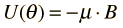The magnetic dipole moment associated with the orbital angular momentum is given by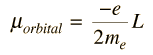For a magnetic field in the z-direction this gives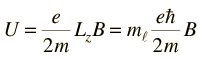Considering the quantization of angular momentum, this gives equally spaced energy levels displaced from the zero field level byThis displacement of the energy levels gives the uniformly spaced multiplet splitting of the spectral lines which is called the Zeeman effect.

The magnetic field also interacts with the electron spin magnetic moment, so it contributes to the Zeeman effect in many cases. The electron spin had not been discovered at the time of Zeeman's original experiments, so the cases where it contributed were considered to be anomalous. The term "anomalous Zeeman effect" has persisted for the cases where spin contributes. In general, both orbital and spin moments are involved, and the Zeeman interaction takes the formThe factor of two multiplying the electron spin angular momentum comes from the fact that it is twice as effective in producing magnetic moment. This factor is called the spin g-factor or gyromagnetic ratio. The evaluation of the scalar product between the angular momenta and the magnetic field here is complicated by the fact that the S and L vectors are both precessing around the magnetic field and are not in general in the same direction. The persistent early spectroscopists worked out a way to calculate the effect of the directions. The resulting geometric factor gL in the final expression above is called the Lande g factor. It allowed them to express the resultant splittings of the spectral lines in terms of the z-component of the total angular momentum, mj.

The above treatment of the Zeeman effect describes the phenomenon when the magnetic fields are small enough that the orbital and spin angular momenta can be considered to be coupled. For extremely strong magnetic fields this coupling is broken and another approach must be taken. The strong field effect is called the Paschen-Back effect.

Index

Schrodinger equation concepts

 HyperPhysics***** Quantum Physics R Nave
Go Back

# Hydrogen Zeeman Example

The Zeeman effect for the hydrogen atom offered experimental support for the quantization of angular momentum which arose from the solution of the Schrodinger equation.Index

Schrodinger equation concepts

 HyperPhysics***** Quantum Physics R Nave
Go Back

# "Anomalous" Zeeman Effect

While the Zeeman effect in some atoms (e.g., hydrogen) showed the expected equally-spaced triplet, in other atoms the magnetic field split the lines into four, six, or even more lines and some triplets showed wider spacings than expected. These deviations were labeled the "anomalous Zeeman effect" and were very puzzling to early researchers. The explanation of these different patterns of splitting gave additional insight into the effects of electron spin. With the inclusion of electron spin in the total angular momentum, the other types of multiplets formed part of a consistent picture. So what has been historically called the "anomalous" Zeeman effect is really the normal Zeeman effect when electron spin is included.

 "Normal" Zeeman effect This type of splitting is observed with hydrogen and the zinc singlet.This type of splitting is observed for spin 0 states since the spin does not contribute to the angular momentum. "Anomalous" Zeeman effect When electron spin is included, there is a greater variety of splitting patterns.Zeeman interaction Sodium Zeeman effect
Index

Atomic Structure Concepts

Reference
Richtmyer, et al.
Ch. 18

 HyperPhysics***** Quantum Physics R Nave
Go Back

# The Electron Spin g-factor

When the Zeeman effect was observed for hydrogen, the observed splitting was consistent with an electron orbit magnetic moment given by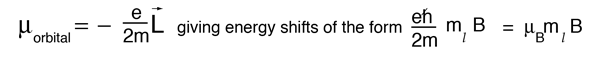where the splittings followed the z-component of angular momentum and the selection rules explained why you got a triplet of closely-spaced lines for the 3 -> 2 transition of hydrogen. But when the effects of electron spin were discovered by Goudsmit and Uhlenbeck, they found that the observed spectral features were matched by assigning to the electron spin a magnetic momentwhere g is approximately 2.

More precise experiments showed that the value was slightly greater than 2, and this fact took on added importance when that departure from 2 was predicted by quantum electrodynamics. In the experimental measurement of the Lamb shift, the value of g has been determined to be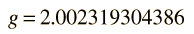and is in precise agreement with the calculated values.

Index

Schrodinger equation concepts

 HyperPhysics***** Quantum Physics R Nave
Go Back